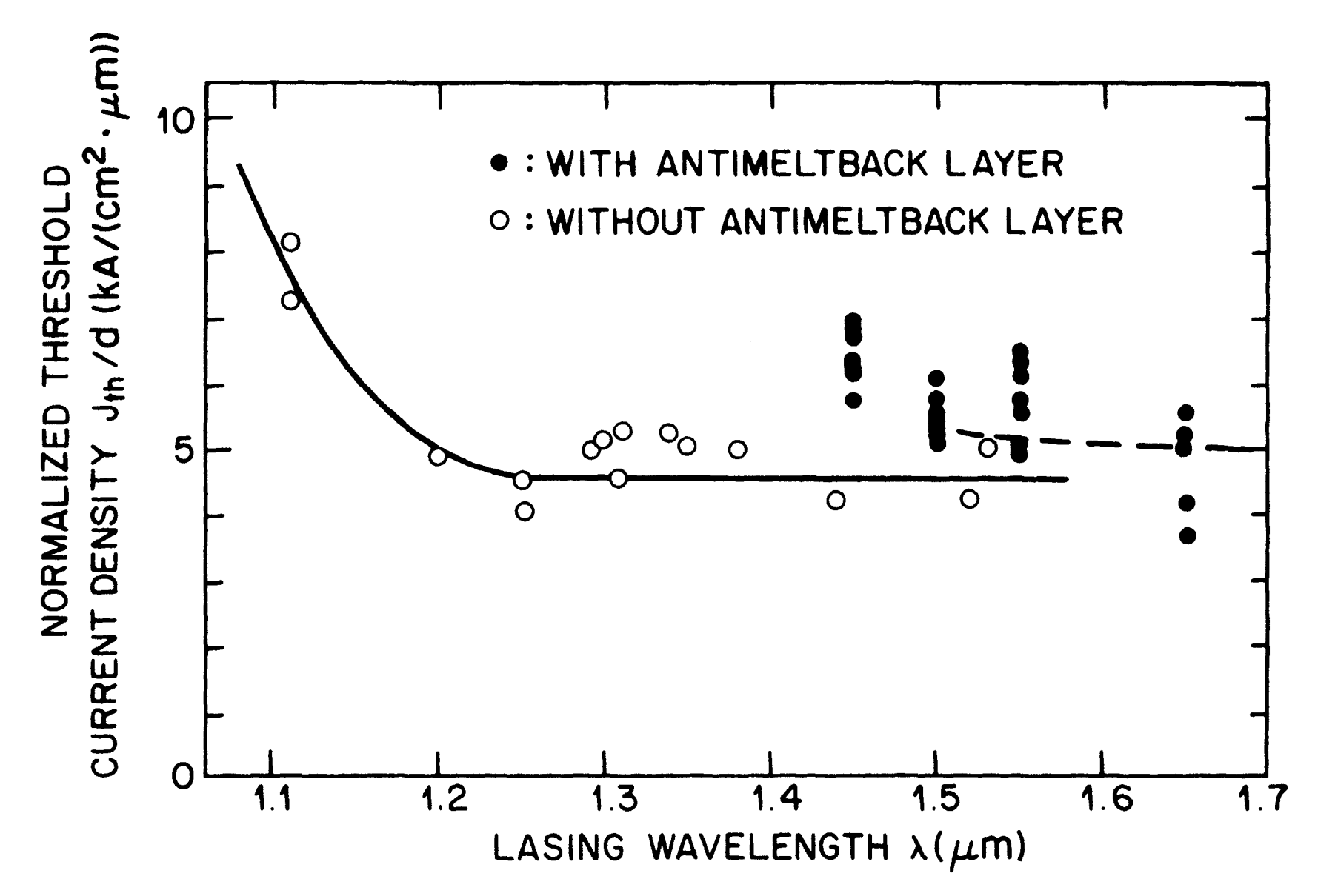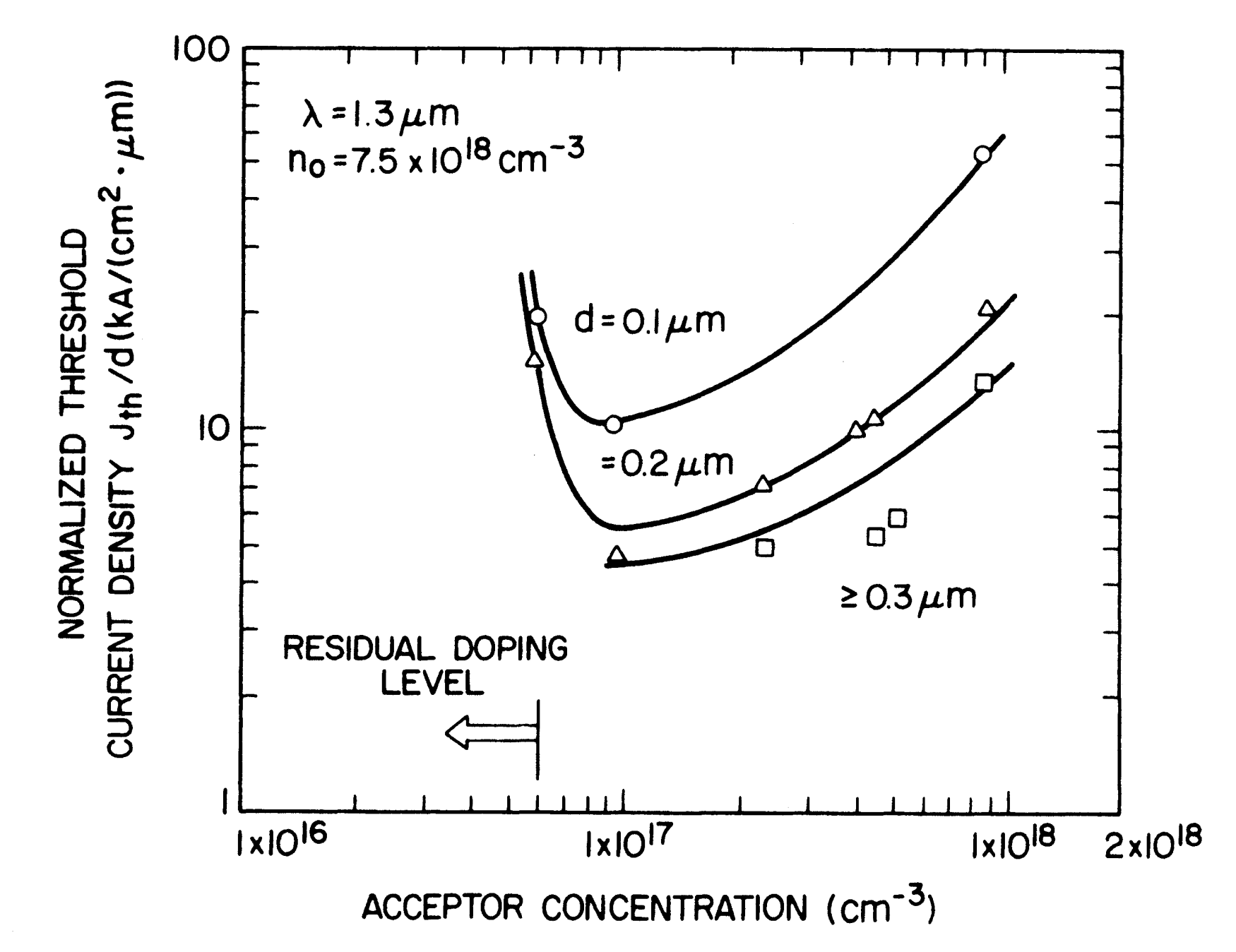This is continuation from the previous tutorial - what is strained-layer epitaxy.

## Introduction

Semiconductor lasers operating in the wavelength range of 1.1-1.65 μm can be fabricated using the InGaAsP quaternary material which has been grown lattice-matched on an InP substrate. Room-temperature continuous operation of InGaAsP-InP double-heterostructure lasers was first reported in 1976.

Since then, a large number of laser structures have been developed guided by the performance requirements of specific applications. The next few tutorials discuss different InGaAsP laser structures with particular emphasis on their performance in terms of the light-current characteristics, the threshold current, and the threshold current's temperature dependence.

Other performance characteristics that make a laser structure suitable as a source for high-speed digital lightwave transmission systems are discussed in detail in a later tutorial series.

In a double-heterostructure laser, the optical mode is confined perpendicular to the junction plane because the cladding layers have a lower index of refraction compared with that of the active region. For a stable fundamental-mode operation with a low threshold current, additional confinement of the optical mode along the junction plane is required.

In the absence of this lateral-mode confinement (which may be induced by a region of well-defined optical gain or a refractive index step), the laser behaves as a broad-area laser with a threshold current density of $$\sim1\text{ kA/cm}^2$$; i.e., a threshold current of $$\sim1\text{ A}$$ is required for a laser of cross section 250 μm x 380 μm. Such a high threshold current would limit the usefulness of semiconductor injection lasers.

The laser structures described in the next few tutorials are essentially different ways of realizing the lateral-mode confinement; the eventual aim is to obtain a laser structure with a low threshold current that can operate in the fundamental lateral mode up to high powers. State-of-the-art InGaAsP lasers emitting near 1.3 μm generally have threshold currents in the range of 10-15 mA at $$20^\circ\text{C}$$.

As discussed in the waveguide modes in semiconductor lasers tutorial, the laser structures can in general be classified into two groups, gain-guided and index-guided.

In the gain-guided structure, the width of the optical mode along the junction plane is mainly determined by the width of the optical gain region, which in turn is determined by the width of the current-pumped region (typically in the range of 5-10 μm).

In index-guided lasers, a narrow central region of relatively higher refractive index in the junction plane confines the lasing mode to that region. The index-guided lasers can be divided into two general subgroups, weakly index-guided and strongly index-guided lasers.

In weakly index-guided lasers, the active region is continuous and the effective index discontinuity is provided by a cladding layer of varying thickness. Depending on the specific design, these structures are known under various names, such as rib waveguide, ridge waveguide, plano-convex waveguide, and channeled-substrate planar waveguide.

By contrast, the strongly index-guided lasers employ a buried heterostructure. In these lasers, the active region is bounded by low-index, epitaxially grown layers both along and normal to the junction plane.

The lateral index difference is $$\sim0.01$$ for weakly index-guide lasers and $$\sim0.2$$ for strongly index-guided, buried-heterostructure lasers.

Gain-guided lasers are easier to fabricate than index-guide lasers. However, gain-guided lasers exhibit higher threshold currents than do index-guide lasers.

Typical threshold currents of gain-guided lasers are int he range of 100-150 mA compared with 10-15 mA for buried-heterostructure lasers.

The fabrication of strongly index-guided lasers generally requires either a single epitaxial growth over nonplanar surfaces or two epitaxial growths and, in addition, careful attention to processing.

In spite of these difficulties in fabrication, their superior performance characteristics - low threshold current, stable fundamental-mode operation at high powers, and good high-speed modulation characteristics - make them a prime candidate for high-performance applications.

In particular, their use as a source in lightwave transmission systems is highly attractive. In fact, the development of various types of strongly index-guided laser structures over the last years principally has been carried out using InGaAsP. This is a material of choice for long-haul lightwave transmission system applications.

Weakly index-guided lasers are easier to fabricate than strongly index-guided lasers but generally have a higher threshold current (~ 50 mA). These lasers can be a useful alternative to strongly index-guided lasers in less demanding applications.

The laser structures described in the following few tutorials are generic, in principle, to any material system, although we focus our attention on the use of InGaAsP. The InGaAsP alloy can be grown lattice-matched on InP and can emit light in the wavelength of 1.1-1.65 μm.

A useful figure of merit, indicative of the "quality" of a semiconductor laser material, is the broad-area threshold current density.

A broad-area laser does not imply any scheme for current confinement. The processing of these devices is relatively simple. Most of the early laser work using InGaAsP was carried out using broad-area structures grown by liquid-phase epitaxy (LPE) on an n-type InP substrate.

The as-grown wafer, typically 250-400 μm thick, is thinned down to a thickness of 75-100 μm, and metallic contacts are deposited on the substrate side (typically AuSn or AuGe alloy) and the epitaxially grown side (typically CrAu or AuZn alloy).

The thinning of the wafer is necessary to facilitate cleaving along a crystallographic plane. The cleaved planes [typically (110)] form the mirror of the laser cavity. The other sides are saw-cut.

Figure 5-1 shows a schematic of a broad-area laser chip, together with typical device dimensions.Figure 5-1. Schematic illustration of a typical broad-area laser chip for a 1.3-μm InGaAsP double-heterostructure laser.

The threshold current density $$J_\text{th}$$ depends on the confinement of the optical mode and hence varies with the thickness $$d$$ of the active region [see Equations (2-6-2) and (2-6-3) in the emission characteristics of semiconductor lasers tutorial].

Figure 5-2 shows experimental data for $$J_\text{th}$$ as a function of $$d$$ for 1.3-μm InGaAsP-InP double-heterostructure lasers.Figure 5-2. Measured threshold current density at room temperature as a function of the active-layer thickness for 1.3-μm InGaAsP double-heterostructure lasers.

We note that $$J_\text{th}$$ is minimum for an optimum value of $$d$$. The rapid increase for $$d$$ when $$d\lt0.1$$ μm is due to the loss in mode confinement for thin active layers.

The measured threshold current density varies linearly with $$d$$ for thickness greater than 0.2 μm. Thus $$J_\text{th}/d$$ is a useful universal parameter for the characterization of lasers emitting at different wavelengths or lasers fabricated using different material systems.

The quantity $$J_\text{th}/d$$ equals the nominal current density defined by Equation (3-2-44) [refer to the radiative recombination in semiconductors tutorial] in the absence of nonradiative recombination mechanisms.

Figure 5-3 shows the experimental data for $$J_\text{th}/d$$ for InGaAsP lasers emitting at different wavelengths.Figure 5-3. Experimental data for $$J_\text{th}/d$$ for broad-area InGaAsP lasers emitting at different wavelengths.

For lasers emitting at wavelengths greater than 1.5 μm it is often necessary to grow an additional layer (called the antimeltback layer) on top of the active layer to prevent melting back of the active region during the LPE growth of the p-InP cladding layer. The band gap of the antimeltback layer is intermediate to that of surrounding layers.

Figure 5-3 shows the data for lasers with and without antimeltback layers. In all cases the average value of $$J_\text{th}/d$$ is $$\sim5\text{ kA}/(\text{cm}^2\cdot\text{μm})$$ and is found to be independent of wavelength in the range of 1.2-1.65 μm. This value is comparable to the values reported for AlGaAs lasers.

Various factors that can affect the threshold current density of a broad-area laser have been theoretically discussed in the emission characteristics of semiconductor lasers tutorial.

One of the factors is the carrier concentration in the active region. It is generally accepted that increased p doping of the active region can increase the threshold current.

The effect is partly due to an increase in the nonradiative Auger recombination rate and partly due to an increase in optical absorption.

The former manifests itself in a decrease in the carrier lifetime [see Equation (2-6-3) in the emission characteristics of semiconductor lasers tutorial], and the latter causes a decrease in the external differential quantum efficiency [see Equation (2-6-11) in the emission characteristics of semiconductor lasers tutorial].

In the fabrication of InGaAsP-InP lasers, the active layer si not intentionally doped. However, during LPE of the n-InP cladding layer that is usually doped using Zn, a diffusion of Zn into the active layer takes place and makes it p type.

Experimental data for the observed threshold current density as a function of the acceptor concentration of the p-InP cladding layer are shown in Figure 5-4 for three different thicknesses of the active layer.Figure 5-4.  Measured threshold current density of 1.3-μm InGaAsP-InP broad-area lasers as a function of the acceptor concentration in the p-InP cladding layer. The donor concentration is kept constant.

The donor concentration of the n-InP layer in these experiments was $$7.5\times10^{18}\text{ cm}^{-3}$$. Figure 5-4 shows that thicker active layers are less affected than thinner layers in the range of $$10^{17}-10^{18}\text{ cm}^{-3}$$. The increase of threshold current for cladding-layer doping levels less than $$10^{17}\text{ cm}^{-3}$$ is probably due to a displacement of the p-n junction into the p-cladding layer during growth.

The threshold current density of a broad-area laser also depends on the cavity length $$L$$. This dependence can be seen from Equations (2-6-2) [refer to the emission characteristics of semiconductor lasers tutorial] and (2-3-10) [refer to the threshold condition and longitudinal modes of semiconductor lasers tutorial].

For long cavities, the distributed mirror loss $$\alpha_\text{m}$$ is small compared to the internal loss $$\alpha_\text{int}$$. Itaya et al. have reported a threshold current density of $$770\text{ A/cm}^2$$ for a cavity length of 1500 μm in an InGaAsP laser emitting at 1.3 μm. More recently, values as low as $$\sim500\text{ A/cm}^2$$ have been observed with design optimization for 1.3-μm InGaAsP lasers of about 400 μm in length.

The next tutorial discusses about gain-guide lasers.